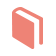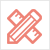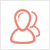﻿ 留学资讯-Global Positioning System (GPS) project-51Due留学教育

#### 服务承诺资金托管原创保证实力保障24小时客服使命必达

#### 关于我们

51Due提供Essay，Paper，Report，Assignment等学科作业的代写与辅导，同时涵盖Personal Statement，转学申请等留学文书代写。51Due将让你达成学业目标51Due将让你达成学业目标51Due将让你达成学业目标51Due将让你达成学业目标

#### 名企实习积累工作经验多元化文化交流专业实操技能建立人际资源圈

# Global Positioning System (GPS) project

2020-06-04 来源: 51Due教员组 类别: 留学资讯Global Positioning System (GPS) project

Mathematical Approach-

Satellite

Beginning with the approach towards our satellite, conversion programs were written in order to convert angles from degrees, minutes, and seconds to radians, and vice versa. Next, we used the formula derived from exercise #4 from equations; (19), (18), (17), (14), and (13) in the project homework assignment. This gives us a formula from converting position and general time t as given in [ tV  Ψd  Ψm  Ψs  NS  λd  λm  λs  EW  h (10)] into Cartesian coordinates. Since the main purpose for each of our programs is to take in standard input, and out standard output, we used the information gained in exercise 6 to convert the data from Cartesian coordinates into form (10). In order to complete this task we used and added some additional parameters to (20).

 h = sqrt(x2 + y2 +z2) – R {arctan (z/sqrt(x2 + y2)) Ψ =   {π/2          {-π/2 For x2 + y2 ≠ 0 For x = y = 0, z > 0 For x = y = 0, z < 0 {π/2          {-π/2 λ =    {arctan (y/x)          {π + arctan(y/x)          {2π + arctan(y/x) For x = 0, y > 0 For x = 0, y < 0 For x > 0, y > 0 For x < 0 For x > 0, y < 0

 s above horizon ßà xTs > xTx

We were then compelled to find a condition set to assure us the satellites used for our data were above the horizon. Given in Cartesian coordinates of the following equation (31), we figure x being a point on earth and s, in space, this tells us whether s viewed from x is in fact above the horizon.

 t0 = tV - ǁxs(tV)-xVǁ2/c

Our final part in solving the satellite, is to compute our ts and xs, being time and position of the satellite respectively. We continued following the iteration from exercise 9 and solving t0 in the form (40).

As mentioned in the project assignment, we have 4 unknowns with coordinates of location and time, which leaves us a range within c(tS – tV), where we do not know tV. For our mathematical approach in solving our system of 4 nonlinear equations, we chose to follow exercise 14, and solving thru Newton’s method, the nonlinear system obtained by the Least Squares approach. Due to the possibility of failure in solving this non-linear system, we chose our initial points as such: for the receiver, denote geo0 and geo1 be last two geographic coordinates of vehicle's solution. Let d_geo be the difference of geo1 and geo0 and geo = d_geo + geo1.  Then init_t = t_s+.08 and init_x = geography2cartesian(init_t, geo). We used .08s for the addition to t_s, because it is experimentally the average time that the signal takes to go to the vehicle. Also, let it be known that the init_x is approximated by assuming the velocity of the vehicle, constant over a short period.

Directions/How to use-

Our programming language consisted of using C++.

Satellite takes geographic data from standard input [ tV  Ψd  Ψm  Ψs  NS  λd  λm  λs  EW  h (10)] and generates data from satellites above the horizon in the form [iS tS xS] (26). The receiver takes data in form (26), and prints to standard output in (10). Both the satellite and receiver use data.dat parameters. These programs can be used by giving a command like:

cat bm.dat | java vehicle | ./satellite | ./receiver

Lesson Learned-

We noticed in derivatives that there could have been problems in diving the Ni = ǁxS - xǁ2, since Ni is large, we avoided dividing by zero. From this, we learned that we should divide by larger numbers, but if in another project Ni was small, we would know to modify the problem or equation to divide by a reasonably large number to avoid having to divide by zero.

Other worth mentioning-

The project required that the accuracy of the GPS be within .01m. But to make it safe, the receiver was programmed to have .0001m as the condition of convergence. Also, the accuracy of time is c times of length accuracy, thus making both items at a higher degree of accuracy.

Additionally, we have also tried to solve satellites by using Newton’s method (form 38) and solve receiver by taking first 4 satellites and applying Newton’s method (exercise 13). It turns out that least square method for receiver and Newton’s method for satellite requires more actual initial point.

51due留学教育原创版权郑重声明：原创优秀代写范文源自编辑创作，未经官方许可，网站谢绝转载。对于侵权行为，未经同意的情况下，51Due有权追究法律责任。主要业务有essay代写、assignment代写、paper代写、作业代写服务。

51due为留学生提供最好的作业代写服务，亲们可以进入主页了解和获取更多代写范文提供作业代写服务，详情可以咨询我们的客服QQ：800020041。